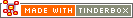^if( condition )^Code Type: Conditional Mark-up Code Scope of Action: n/a

^if( condition )^

Test if the condition is true, then includes everything from the ^if statement to the ^else^ or the ^endif^. If the else/endif mark-up is omitted the text following the closing ) bracket of the condition is returned. These are functionally the same:

• Color=^if(^descendants^)^red
• Color= ^if(^descendants^)^red^endif^

Whilst the usual way to test for the existence of an object or group remains

^if(^exists(child)^)^

... the shorter test syntax

^if(^children^)^

works as well — i.e. if a note has any descendants, then ^children^ is true.

Note there is no 'else if' branching syntax; for such tests nest if statements.

The export element ^if^ accepts agent query expressions in the condition. For example,

`^if(Name(tbx))^`

...is true if the note's title contains the string "tbx",

`^if(!Name(tbx))^`

...is true if the note's title does not contain the string "tbx", and

`^if(Width>5)^`

...is true if the note's width exceeds 5.0

For the first two examples above the value matched can be an actual string or a regular expression (see more on this).

In the ^if^ condition, the agent query is always evaluated in the context of this note.

A slightly different if syntax should used in Actions and Rules: if(query){action list}else{action list}. This syntax can only be used internally in Actions and Rules. In such context, either if syntax may be used but the ^if version, with carets, is the only one that may be used in export templates.

 Up: Scope n/a Previous: ^firstWord( data )^ Next: ^lastWord( data )^

[Last updated: 14 Dec 2009, using v5.0]